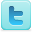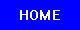﻿ Graham's Laws: Diffusion and Effusion of Gases - Experiments
Graham's Laws: Diffusion and Effusion of Gases
K-12 Experiments & Background Information
For Science Labs, Lesson Plans, Class Activities & Science Fair Projects
For Middle and High School Students & Teachers

Gas Diffusion and Effusion Experiments

Graham's Laws Background

## Definitions

Diffusion - The spread of particles through random motion from regions of higher concentration to regions of lower concentration.

Effusion - The process in which individual molecules flow, through a hole without collisions between molecules, into a vacuum.

## Graham's Laws

Graham's law, also known as Graham's law of effusion, was formulated by Scottish physical chemist Thomas Graham. Graham found experimentally that the rate of effusion of a gas is inversely proportional to the square root of the mass of its particles. This formula can be written as: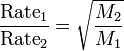where:

Rate1 is the rate of effusion of the first gas (volume or number of moles per unit time).

Rate2 is the rate of effusion for the second gas.

M1 is the molar mass of gas 1

M2 is the molar mass of gas 2.

Graham's law states that the rate of effusion of a gas is inversely proportional to the square root of its molecular weight. Thus, if the molecular weight of one gas is four times that of another, it would diffuse through a porous plug or escape through a small pinhole in a vessel at half the rate of the other. A complete theoretical explanation of Graham's law was provided years later by the kinetic theory of gases. Graham's law provides a basis for separating isotopes by diffusion — a method that came to play a crucial role in the development of the atomic bomb.

Graham's law is most accurate for molecular effusion which involves the movement of one gas at a time through a hole. It is only approximate for diffusion of one gas in another or in air, as these processes involve the movement of more than one gas.

Graham's law states that the rate at which gas molecules diffuse is inversely proportional to the square root of its density. Combined with Avogadro's law (i.e. since equal volumes have equal number of molecules) this is the same as being inversely proportional to the root of the molecular weight.

## History

Graham's research on the diffusion of gases was triggered by his reading about the observation of German chemist Johann Döbereiner that hydrogen gas diffused out of a small crack in a glass bottle faster than the surrounding air diffused in to replace it. Graham measured the rate of diffusion of gases through plaster plugs, through very fine tubes, and through small orifices. In this way he slowed down the process so that it could be studied quantitatively. He first stated the law as we know it today in 1831. Graham went on to study the diffusion of substances in solution and in the process made the discovery that some apparent solutions actually are suspensions of particles too large to pass through a parchment filter. He termed these materials colloids, a term that has come to denote an important class of finely divided materials.

At the time Graham did his work the concept of molecular weight was being established, in large part through measurements of gases. Italian physicist Amadeo Avogadro had suggested in 1811 that equal volumes of different gases contain equal numbers of molecules. Thus, the relative molecular weights of two gases are equal to the ratio of weights of equal volumes of the gases. Avogadro's insight together with other studies of gas behaviour provided a basis for later theoretical work by Scottish physicist James Clerk Maxwell to explain the properties of gases as collections of small particles moving through largely empty space.

Perhaps the greatest success of the kinetic theory of gases, as it came to be called, was the discovery that for gases, the temperature as measured on the Kelvin (absolute) temperature scale is directly proportional to the average kinetic energy of the gas molecules. The kinetic energy of any object is equal to one-half its mass times the square of its velocity. Thus, to have equal kinetic energies, the velocities of two different molecules would have to be in inverse proportion to the square roots of their masses. The rate of effusion is determined by the number of molecules entering an aperture per unit time, and hence by the average molecular velocity. Graham's law for diffusion could thus be understood as a consequence of the molecular kinetic energies being equal at the same temperature.

## Example

Let gas 1 be H2 and gas 2 be O2.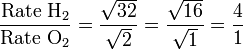Therefore, hydrogen molecules effuse four times faster than those of oxygen.

Graham's Law can also be used to find the approximate molecular weight of a gas if one gas is a known species, and if there is a specific ratio between the rates of two gases (such as in the previous example). The equation can be solved for either one of the molecular weights provided the subscripts are consistent.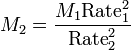Graham's law was the basis for separating 235U from 238U found in natural uraninite (uranium ore) during the Manhattan project to build the first atomic bomb. The United States government built a gaseous diffusion plant at the then phenomenal cost of \$100 million in Clinton, Tennessee. In this plant, uranium from uranium ore was first converted to uranium hexafluoride and then forced repeatedly to diffuse through porous barriers, each time becoming a little more enriched in the slightly lighter 235U isotope.

Source: Wikipedia (All text is available under the terms of the GNU Free Documentation License and Creative Commons Attribution-ShareAlike License.)

Science Fair Projects Resources
Physics Science Fair Books